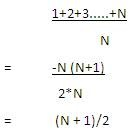Sequential Search is the most natural searching method. In this method, the searching begins with searching every element of the list till the required record is found. It makes no demands on the ordering of records. It takes considerably amount of time and is slower.

# Sequential Search Algorithm

This represents the algorithm to search a list of values of to find the required one.

```INPUT: List of size N. Target value T
OUTPUT: Position of T in the list I
BEGIN

1. Set FOUND to false
Set I to 0

2. While (I<=N) and (FOUND is false)
If List [I] = T
FOUND = true
Else
I=I+1
END

3. If FOUND is false
T is not present in List.
END
```

### Analysis of Sequential Search

Whether the sequential search is carried out on lists implemented as arrays or linked lists or on files, the criterial part in performance is the comparison loop step 2. Obviously the fewer the number of comparisons, the sooner the algorithm will terminate.

The fewest possible comparisons = 1. When the required item is the first item in the list. The maximum comparisons = N when the required item is the last item in the list. Thus if the required item is in position I in the list, I comparisons are required. Hence the average number of comparisons done by sequential search is (N+1)/2Sequential search is easy to write and efficient for short lists. It does not require sorted data. However it is disastrous for long lists. There is no way of quickly establishing that the required item is not in the list or of finding all occurrences of a required item at one place. We can overcome these deficiencies with Binary search.

### Example- Program to search for an item using linear search.

```#include < stdio.h >

/* Search for key in the List */
int seq_search(int key, int a[], int n)
{
Int I;
for (i = 0; i < n; i++)
{
If(a[i] == key) return i + 1
}
return 0;
}

void main()
{
int I, n, key, pos, a;
printf("Enter the value of n");
scanf("%d", & n);
printf("Enter n valuesn");
for (i = 0; i < n; i++)
scanf("%d", & a[i]);

printf("Enter the item to be searched");
scanf("%d", & key);

pos = seq_search(key, n, a);
if (pos == 0)
printf("Search unscccessful n");
else
printf("key found at position = %d n", pos);
}
```